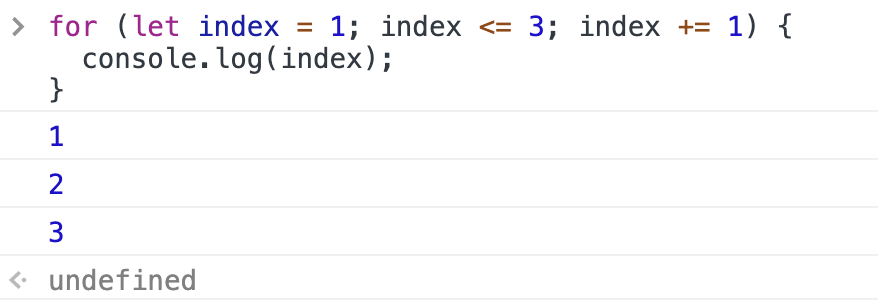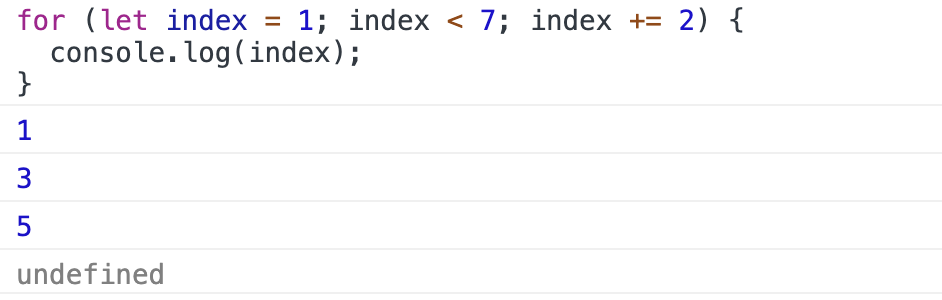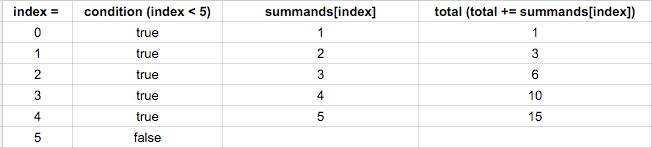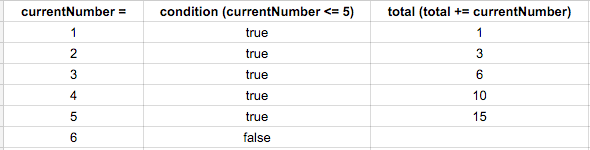Lesson Wednesday

`Array.prototype.forEach()` is a relatively new addition to JavaScript. Under the hood, it uses what's known as a `for` loop.

In this lesson, we'll cover how a `for` loop works. Then, in the next lesson, we'll discuss when to favor `Array.prototype.forEach()` and when to stick with a `for` loop. Finally, we'll add a function to our text analyzer application that uses a `for` loop.

## `for` Loops

Let's take a look at a basic example of a `for` loop. We'll see what it prints to the DevTools console and then we'll go over all of the parts of the loop.

Here's a loop that logs the value of `index`. We'll be talking about what the `index` is in just a moment.

``````> for (let index = 1; index <= 3; index += 1) {
console.log(index);
}
``````

Let's see what this logs to the DevTools console.As we can see, the `index` is an incrementing number. We can also see that the return of a `for` loop is `undefined`, just like it is with `Array.prototype.forEach()`. (This isn't surprising — remember, under the hood, `Array.prototype.forEach()` is using a `for` loop.)

Here is the syntax of a `for` loop. This is pseudocode, which means it isn't written like JavaScript; it's written in plain English to explain how it works.

``````// Pseudocode alert!

for (let index = startingValue; index <= endingValue; index += increment ) {
// Code that should run each time through the loop!
}
``````

Now let's break this down further.

• The `for` statement takes three parameters: initialization, condition, and final expression.

• The initialization parameter is the starting value of the loop. In the example above, we called it `startingValue`. `index` is a variable we initialize with our starting value. Very often, that starting value is `0` or `1`, but depending on your use case, it could be any number.

• The initialization parameter is executed before the loop runs. Because `index` is a variable that changes, we need to use `let` with it. `const` will not work. If we omit `let`, we are accidentally scoping the value of `index` globally — a very bad idea, especially if there are other `for` loops using `index` in the codebase.
• The condition parameter tells the loop when to stop running — or more accurately when to keep running. The loop will stop when the condition is no longer true. In our first example of a loop, we set the condition parameter to `index <= 3`. This means that when the index variable is NOT less than or equal to 3, the loop will stop. Until then, it will keep going. The condition is evaluated every time the loop runs. We can use other operators to denote this depending on how our loop works, including `<`, `>`, and `>=`.

• The final expression parameter changes the value of `index` by incrementing or decrementing it for every iteration of the loop. In our example, the index variable will be increased by 1 after each time the loop is executed.

• Each time the loop runs, the code within the curly brackets will run. In our first example of a loop, we log the value of `index` with `console.log(index);`.

• Note that each parameter has a semicolon as a separator. A `for` loop will not work without these separators.

In our first example of a loop, we specify that the `index` will start at 1 and end at 3. Each time through the loop, the `index` will increment by 1. The loop will run 3 times before it reaches 3 and ends.

Now it's time for a bit of a brainteaser — how many times will the `for` loop below run and what will the value of `index` be in the console each time it runs? See if you can figure it out before running the code in the console.

``````for (let index = 1; index < 7; index += 2) {
console.log(index);
}
``````

Now let's take a look at the code:There are a couple of little gotchas here. First, we are incrementing by 2, not 1. That means the value of index will increase like this... `1`, `3`, `5`... The most common increment and decrement is 1, but it's important to see exactly what's happening with the final expression parameter.

The other gotcha is where it stops. Did you think the loop would run until the `index` reached `7`? If so, you're not alone. In the example above, we changed the comparison operator we use in the condition parameter from `<=` to `<`. If we'd used `<=` instead, the loop would've run one more time and the `index` would've ended at `7`.

So as you can see, `for` loops can be tricky! We'll discuss that further in the next lesson.

### Using a `for` Loop with an Array

Now that we've dissected a very basic `for` loop, how can we actually use it with a collection like an array? Unlike `Array.prototype.forEach()`, a `for` loop isn't called on an array.

Remember how we doubled all the values of an array with `Array.prototype.forEach()` and then saved those values in a new array? Here's how we did it:

``````> const array = [0,1,2,3,4,5];
> let doubledArray = [];
> array.forEach(function(element) {
doubledArray.push(element * 2);
});
> doubledArray;
(6) [0, 2, 4, 6, 8, 10]
``````

Now let's do the same with a `for` loop.

``````> const array = [0,1,2,3,4,5];
> let doubledArray = [];
> for (let index = 0; index < array.length; index +=1) {
doubledArray.push(array[index] * 2);
}
> doubledArray;
(6) [0, 2, 4, 6, 8, 10]
``````

Some of this should look familiar — we don't change the `array` we loop over. We also need to initialize a new array called `doubledArray` that we'll push the doubled values into — just like we did when using `Array.prototype.forEach()`.

Now let's look at the `for` loop. Note that the `index` starts at 0, not 1. If we are looping through an entire array, the index starts at 0 because the index of the first element in an array is 0.

Next, we specify that the condition parameter is `index < array.length;`. We could just as easily have written this as `index <= array.length - 1`. Remember, because the index of the first element in an array is 0 instead of 1, the index of the final element in an array will always be one less than the array's length.

Finally, we increment the index by 1 each time an iteration through the loop is finished. So why do we want to increment by 1 each time through? Let's look at the code in the loop itself to see why:

``````doubledArray.push(array[index] * 2);
``````

To access a value from our original `array`, we need to use bracket notation. The first value of `array` is `array`, the second value is `array`, and so on. Well, that matches our index perfectly! That's exactly why we start with an index of `0` when we use `for` loops to work with arrays and then increment by `1` until we reach one less than the length of the array.

We are going to look at more examples in a moment to solidify `for` loops further. But first, an aside to talk about the risk of dreaded OBOEs (off-by-one errors).

### OBOEs in `for` Loops

In general, whenever we are iterating over every element in an array, we should use `Array.prototype.forEach()` instead of `for`. It's not just that it's easier to use. The risk of having an OBOE in a `for` loop is much greater. Let's demonstrate two potential OBOEs based on our doubling example. First, let's imagine that our array doubling example is in a real world application where we figure out the total cost of a certain kind of very important widget. We need to double each element in the array and then add them together in order to figure out the final cost of that widget. (And yes, a more complex algorithm would probably be used in the real world to determine that cost, but bear with us — the exact same errors happen in real world applications.)

Here's the first OBOE. Try it out in the DevTools console:

``````> const array = [0,1,2,3,4,5];
> let doubledArray = [];
> for (let index = 0; index <= array.length; index +=1) {
doubledArray.push(array[index] * 2);
}
> doubledArray;
(7) [0, 2, 4, 6, 8, 10, NaN]
``````

As we can see, we get `NaN` (no a number) as the final array element. Why is this? Well, the final iteration of the loop looks for the value of `array`. There is no element at that index, which returns `undefined`. What happens when you multiply `undefined` by a number? You get `NaN` — because you can't make a number out of it. And what happens when our widget sellers try to calculate the cost of widgets by adding the numbers together? Our formula will return `NaN` — because when you add a real number to `NaN`, it is still `NaN`.

Now let's take a look at an OBOE in the other direction.

``````> const array = [0,1,2,3,4,5];
> let doubledArray = [];
> for (let index = 0; index < array.length - 1; index +=1) {
doubledArray.push(array[index] * 2);
}
> doubledArray;
(5) [0, 2, 4, 6, 8]
``````

As we can see, the final number got lopped off. What changed here is that we did the following `index < array.length - 1`. We are saying the final index should be two less than the length of the array, not one. This error is more insidious — we can't sell widgets that cost `NaN` — and that error will probably get caught quickly. However, this returns an actual number — and if we add all the numbers together, we might be selling widgets at an unsustainable discount. That wouldn't be good!

Lessening the chance of OBOEs is a major reason we should always use `Array.prototype.forEach()` when we need to iterate through every element in an array. That being said, you should still take some time to practice using `for` loops to do the same thing — even though in the long term, it won't be the best practice. It's very important to understand a `for` loop inside and out regardless of how much you'll end up using them!

### Other Examples of `for` Loops

Let's look at a few other examples of `for` loops. In fact, we will just translate all of the loops we made with `Array.prototype.forEach()` into `for` loops. That way, you'll have enough of a foundation to practice creating `for` loops on your own.

We'll start by using a `for` loop to add all the numbers in an array together.

``````> let total = 0;
> const summands = [1, 2, 3, 4, 5];
> for (let index = 0; index < summands.length; index += 1) {
total += summands[index];
}
> total;
15
``````

Try this out in the DevTools console. You'll see it gives the same result as if we'd done it with `Array.prototype.forEach()`.

Here's a corresponding chart that shows what happens each iteration through the loop:Note that when `index` is 0 here, `summands[index]` is 1 because the first element of the array is 1. `index` is just being used here to refer to a specific element of the array by its index position. It's a bit confusing in this case because the elements of the array are also numbers.

Note that we could've created a summands of number 1 through 5 using a `for` loop without creating an array. After all, the `array` variable above just holds an array of sequential numbers.

Here's a version of our sum code that uses a `for` loop but doesn't require an array to loop through. Try it in the DevTools console:

``````> let total = 0;
> for (let currentNumber = 1; currentNumber <= 5; currentNumber += 1) {
total += currentNumber;
}
> total;
15
``````

When the loop begins we initialize our loop variable `currentNumber` to 1. Note that we could have used `index` as the variable name here but `currentNumber` is a bit clearer since we'll be making use of the variable for something other than accessing an array by index. Specifically, we'll be adding `currentNumber` to `total` each time through the loop in addition to using it as an iterator. We then execute our loop as long as `currentNumber` is less than or equal to 5, increasing `currentNumber` by 1 after each run through the loop. Here's a chart showing the steps:The first time through the loop, `currentNumber` is 1, which is <= 5, so we go ahead and execute the statement in the code block: `total += currentNumber`. `total` is now 1 after this first time through the loop. At the end of each time through the loop we're incrementing `currentNumber`, so `currentNumber` is now 2.

The second time through the loop, `currentNumber` is 2, which is still <= 5, so again we execute `total += currentNumber`, setting `total` to 3. We again increment `currentNumber`.

The third time through, `currentNumber` is 3, so `total` becomes 6. And we increment `currentNumber`.

The fourth time through, `currentNumber` is 4, so `total` becomes 10 and then we increment `currentNumber`.

The fifth time through, `currentNumber` is 5, so `total` becomes 15 and we increment `currentNumber`.

The sixth time we try to start going through the loop, `currentNumber` is 6, which is not <= 5, so we're done with the for loop. We then show the user an alert that includes the `total`.

Let's do one more example. This time, we'll recreate the example of appending likable things to a string. For practice, you may want to see if you can recreate the example yourself before looking at the answer. Here's the original using `Array.prototype.forEach()`.

``````> let thingsILike = "I like...";
> const arrayOfThingsILike = ["bubble baths", "kittens", "good books", "clean code"];
> arrayOfThingsILike.forEach(function(thing) {
thingsILike = thingsILike.concat(" " + thing + "!");
});
> thingsILike;
"I like... bubble baths! kittens! good books! clean code!"
``````

Don't look at the translation to a `for` loop just yet... try to write it yourself and test it in the DevTools console!

Here's the version that uses a `for` loop:

``````> let thingsILike = "I like...";
> const arrayOfThingsILike = ["bubble baths", "kittens", "good books", "clean code"];
> for (let i = 0; i < arrayOfThingsILike.length; i+=1) {
thingsILike = thingsILike.concat(" " + arrayOfThingsILike[i] + "!");
}
> thingsILike;
"I like... bubble baths! kittens! good books! clean code!"
``````

Note that we use `i` instead of `index` for our variable name in our example. When you see `for` loops in the wild, you'll most commonly see the variable named `i`. This is short for index — and reflects a desire for maximum conciseness. It's totally fine if you use `i` as well — though it doesn't hurt to use more descriptive names in the short term, especially if it helps you understand what's going on in a loop.

At this point, you're ready to start writing more `for` loops on your own. Once again, be patient with yourself if it takes time to absorb these new concepts. Don't be hard on yourself if you have OBOEs or other errors in your code — they happen to experienced developers, too, and they are an important part of every developer's learning experience.

Finally, you may be wondering why we'd ever favor a `for` loop over an `Array.prototype.forEach()` loop. That's exactly what we'll discuss in the next lesson.

## Terminology

• For loop: The "traditional" way to write loops in JavaScript. Creates a loop without needing to call a method on an array.

• Initialization parameter: Initializes a variable with the denoted value, which usually is a number. This only happens once, when the loop is first triggered. It needs to be initialized with `let`. Usually this variable is called `index` or `i`, but it could be called anything, depending on what the loop is used for and what's most descriptive.

• Condition parameter: Tells the loop under what conditions to continue running the loop. As long as the condition is true, the loop will continue running.

• Final expression parameter: Usually changes the initial value in some way, often by incrementing or decrementing it.

## Examples

Example `for` loop:

``````sfor (let index = 1; index <= 3; index += 1) {
console.log(index);
}
``````

In the code above...

• `let index = 1;` is the initialization parameter.
• `index <=3;` is the condition.
• `index += 1` is the final expression.

Example of using a `for` loop with an array:

``````const languages = ['HTML', 'CSS', 'Javascript'];
for (let index = 0; index < languages.length; index += 1) {
console.log('I love ' + languages[index] + '!');
}
``````